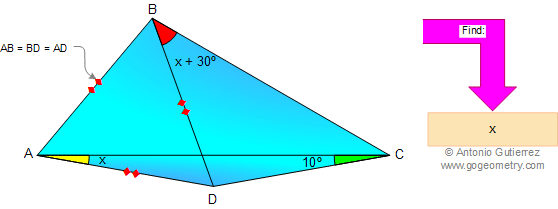# Online Geometry Problem 9. Triangle, Angles, Congruence, 30 Degrees, Auxiliary Lines. Level: High School, Honors Geometry, College, Mathematics Education

< PREVIOUS PROBLEM  |  NEXT PROBLEM >

In the figure below, AB = BD = AD, angle ACD = 10º, angle CAD = x and angle CBD = x + 30º. Find x. Try to use elementary geometry (Euclid's Elements.)